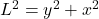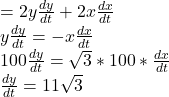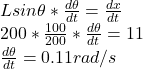## A kite 100 ft above the ground moves horizontally at a speed of 11 ft/s. At what rate is the angle (in radians) between the string and the h

Question

A kite 100 ft above the ground moves horizontally at a speed of 11 ft/s. At what rate is the angle (in radians) between the string and the horizontal decreasing when 200 ft of string have been let out?

in progress 0
4 weeks 2021-08-30T16:00:11+00:00 2 Answers 0 views 0

Explanation:

The solution involves a right angle triangle

Length is z while the horizontal is the height x

X^2+ 100^2=z^2

Taking the derivatives

2x(dx/dt)=Z^2(dz/dt)

Specific moments = Z= 200 ,X= 100sqrt3 and dx/dt= 11

dz/dt= 1100sqrt3/200 = 9.53

Sin a= 100/a

Taking derivatives in terms of t

Cos a(da/dt)=100/z^2 dz/dt

a= 30°

Cos (30°)da/dt= (-100/40000×9.5)

Explanation:

Given  that,

kite is 100 ft high

and moves horizontally at 7 ft/s

Total string let out =200 ft

String length(l), vertical(y) & Horizontal(x) distance of kite will form a right angle triangledifferentiate both sideNow Lcosθ = x

diferentiate